### 4. INFLATIONARY SOLUTIONS TO THE FLATNESS AND HORIZON PROBLEMS

First I will describe the flatness problem and then the inflationary solution to it. Recent measurements of the total density of the Universe find 0.95 <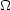o < 1.05 (e.g. Table 1). This near flatness is a problem because the Friedmann Equation tells us that~ 1 is a very unstable condition - like a pencil balancing on its point. It is a very special condition that won't stay there long. Here is an example of how special it is. Equation 16 shows us that (-1 -1)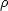R2 = constant. Therefore, we can write,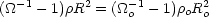(27)

where the right hand side is today and the left hand side is at any arbitrary time. We then have,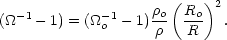(28)

Redshift is related to the scale factor by R = Ro / (1 + z). Consider the evolution during matter-domination where=o(1 + z)3. Inserting these we get,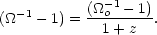(29)

Inserting the current limits on the density of the Universe, 0.95 <o < 1.05 (for which -0.05 < (o-1 -1) < 0.05), we get a constraint on the possible values thatcould have had at redshift z,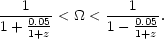(30)

At recombination (when the first hydrogen atoms were formed) z103 and the constraint onyields,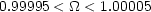(31)

So the observation that 0.95 <o < 1.05 today, means that at a redshift of z ~ 103 we must have had 0.99995 << 1.000005. This range is small...special. However,had to be even more special earlier on. We know that the standard big bang successfully predicts the relative abundances of the light nuclei during nucleosynthesis between ~ 1 minute and ~ 3 minutes after the big bang, so let's consider the slightly earlier time, 1 second after the big bang which is about the beginning of the epoch in which we are confident that the Friedmann Equation holds. The redshift was z ~ 1011 and the resulting constraint on the density at that time was,(32)

This range is even smaller and more special, (although I have assumed matter domination for this calculation, at redshifts higher than zeq ~ 3000, we have radiation domination and=o(1 + z)4. This makes the 1 + z in Eq. 30 a (1 + z)2 and requires that early values ofbe even closer to 1 than calculated here).

To summarize: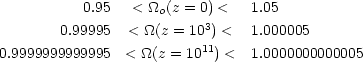(33) (34) (35)

If the Friedmann Equation is valid at even higher redshifts,must have been even closer to one. These limits are the mathematical quantification behind our previous statement that: `If the Universe had started out with a tiny deviation from flatness, the standard big bang model would have quickly generated a measurable degree of non-flatness.' If we assume thatcould have started out with any value, then we have a compelling question: Why shouldhave been so fine-tuned to 1?

Observingo1 today can be compared to a pencil standing on its point. If you walk into a room and find a pencil standing on its point you think: pencils don't usually stand on their points. If a pencil is that way then some mechanism must have recently set it up because pencils won't stay that way long. Similarly, if you wake up in a universe that you know would quickly evolve away from= 1 and yet you find thato = 1 then some mechanism must have balanced it very exactly at= 1.

Another way to state this flatness problem is as an oldness problem. Ifo1 today, then the Universe cannot have gone through many e-folds of expansion which would have driven it away fromo = 1. It cannot be very old. If the pencil is standing on its end, then the mechanism to push it up must have just finished. But we see that the Universe is old in the sense that it has gone through many e-foldings of expansion (even without inflation).

If early values ofhad exceeded 1 by a tiny amount then this closed Universe would have recollapsed on itself almost immediately. How did the Universe get to be so old? If early values ofwere less than 1 by a tiny amount then this open Universe would have expanded so quickly that no stars or galaxies would have formed. How did our galaxy get to be so old? The tiniest deviation from= 1 grows quickly into a collapsing universe or one that expands so quickly that clumps have no time to form.